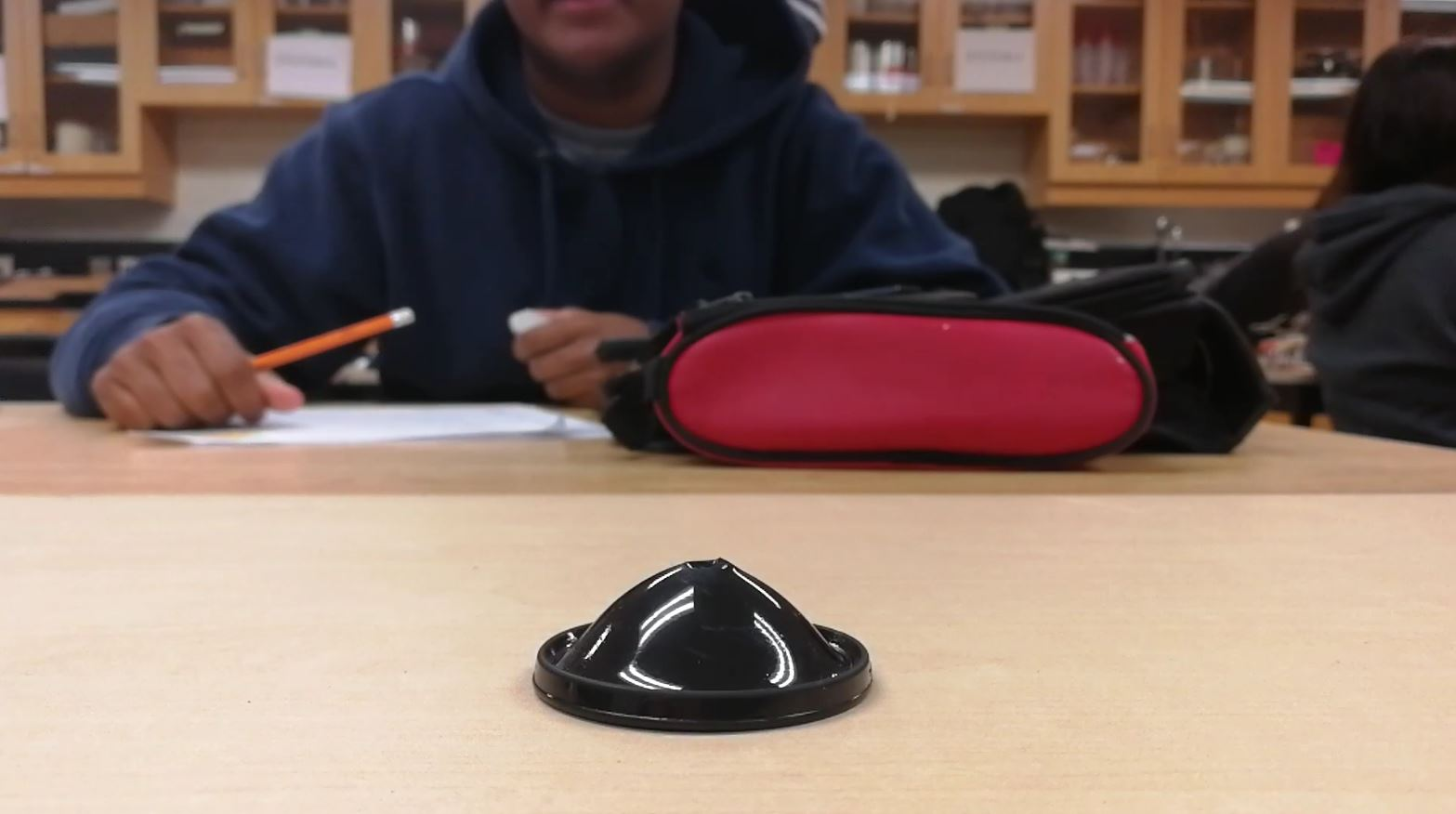## Conservation of Energy

Monday and today, we did a plethora of investigations to further expand our knowledge on the conservation of energy, as well as the transfer of energy!

We rolled marbles down ramps, swung pendulums, and launched poppers! We also explored the flow of energy in a roller coaster. Here’s what I learned:

1) No matter how an object is moved from one height to another, as long as mechanical energy is conserved, the final speed will always be the same. If I were to drop a ball and roll a ball down a ramp from the same starting height to the same end height, they would both have the same final speed. This means that we can ignore what happens in between from one point to another.

What’s important to note is that the way the object is moved does affect the direction of the velocity. So, in the case of the two balls, one would be moving straight downwards, while the other would be moving to the side.

Also, while the end velocity may be the same, a steeper incline will result in a faster conversion from gravitational potential energy to kinetic energy, displacing the marble farther in a shorter amount of time.

2) Mass does not affect the amount of velocity gained from converting gravitational potential energy to kinetic energy. This is because of how mass is relevant in both energy equations: Ek = ½mv2, and Eg = mgh. When you equate the two using the law of conservation of energy, we find that the mass in both equations cancel each other out. We can then derive the equation for velocity:

Ek = Eg

½mv2 = mgΔh

v2 = 2gΔh

v = (2gΔh)1/2

3) If no positive work is done on an object (i.e. no increase in total mechanical energy), it will never be able to reach a height greater than the total mechanical energy divided by the weight of the object. This is primarily due to the conservation of energy, and that gravitational potential energy is given by the equation Eg = mgΔh. From this equation, we get Δh = (Eg)/(mg), and unless there was a change in mechanical energy, the height of the original object cannot be greater than the situation where all mechanical energy is in the form of gravitational potential energy. This is why you can hold a pendulum up to your chin and rest assured that it will not hit you when you let go (provided you don’t put any force on it when you let go).

Conservation of energy is awesome and helps us see how energy changes through math! However, I do have a few questions about what I learned:

1) In the case of a ball rolling down a ramp, does a sharp turn in the incline always result in a perfect change in direction of the velocity? Wouldn’t the ball bounce and lose speed due to the bounce?

2) If an object changes mass while converting between kinetic and gravitational potential energy, how would that affect the motion of the object? Would it somehow be able to achieve a higher height?

Check out this intriguing top that holds gravitational potential energy while spinning. When it stops, it then converts the gravitational potential energy into kinetic energy and turns the other way!

#### After Unit Reflection

##### Investigation of Energy Transformations

In this entire blog post, I did a thorough investigation into energy transformations and the law of conservation of energy through a series of experiments! I made several observations from this investigation. In the first learning point, I found that no matter how the energy is transformed from one form to another (in this case gravitational potential energy to kinetic energy), the final energy will always be equal to the initial energy (as long as no external work was done). In the second learning point, I noted that when transforming kinetic energy to gravitational potential energy (or vice versa), the mass does not affect the final velocity or final height. I also derived the equation for velocity for an object that starts at rest and begins falling. In the third learning point, I learned that due to the conservation of energy, an object at rest cannot attain a greater height without any outside force.